# April Fool's Day Problem of the Day

Apr 01, 2020April Fool’s Day Problem of the Day

Today we are going to take a short break for the typical problem of the day. The first of the April Fool’s Day problems is appropriate for all age groups. The second will test your knowledge of Algebra so is best left to 8th graders and above. I hope you enjoy these brain teaser math tricks.

Problem 1

Write down a three digit number. The first and third digits must differ by more than 1.      735

Reverse the digits to form a second number.        537

Now subtract the smaller number from the larger.   735 - 537 = 198

Now reverse the digits in the answer you got in step three and add it to that number.    198 + 891 = 1089

Multiply the number by 1 million    1089 X 1,000,000 = 1,089,000,000

Subtract 733,361,573 from that number

1,089,000,000 - 733,361,573 = 355,638,427

Under each of the digits in your answer, write the letter which corresponds to it using the following table:

Number Letter

0 Y

1 M

2 P

3 L

4 R

5 O

6 F

7 A

8 I

9 B

Problem 2:

Find which step in the following proof creates the fallacy.

Step 1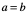Step 2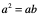Step 3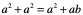Step 4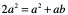Step 5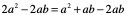Step 6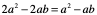Step 7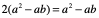Step 8    2 = 1

Problem 1 - April Fool

Problem 2 - Step 8 creates the fallacy because you are dividing both sides by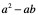which means it cannot be equal to zero but in Step 1 you said a = b somust equal zero.